Previous: 6.1.1.1 Schrödinger Equation Up: 6.1.1 Wave Mechanics Next: 6.1.2 Hydrodynamical Formulation

### 6.1.1.2 Von Neumann Equation

For quantum statistical mechanics the Schrödinger equation gives rise to the von Neumann equation for the density operator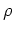(6.12)

where the brackets denote the commutator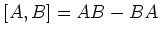(6.13)

of operators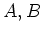. The density operatoris assumed self-adjoint, positive and of trace class. The density matrix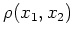is defined by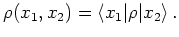(6.14)

For a pure state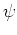the density operator is written in bracket notation as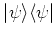(6.15)

and the corresponding matrix in position space is given byThe probabilistic structure is now given by the trace operation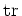. The expectation value of an observable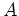becomes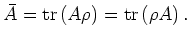(6.16)